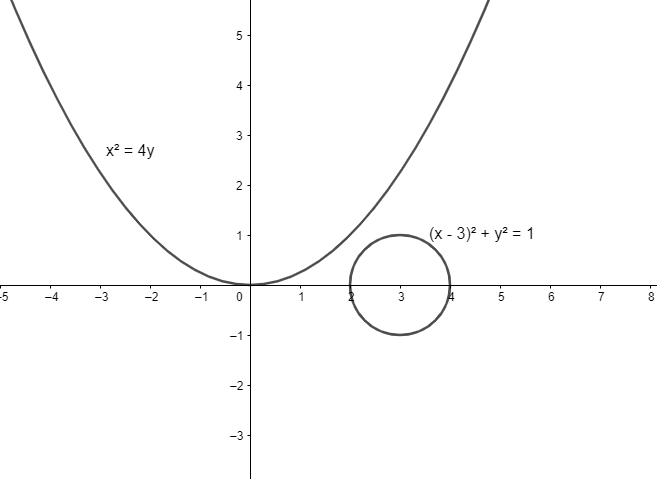Courses
Courses for Kids
Free study material
Offline Centres
MoreLast updated date: 26th Nov 2023
Total views: 383.1k
Views today: 3.83k

# The coordinates of the point on the parabola 24xy which is nearest to the circle${{(x-3)}^{2}}+{{y}^{2}}=1$ are:(a) (0,0)(b) (2,1)(c) (2,1)(d) (4,4)Verified
383.1k+ views
Hint: To find the coordinates of the point on the parabola which has the least
distance from the circle, we will find the distance between the centre of the circle and any
point on the parabola taken in parametric form. Then, we will differentiate that distance and
equate it to zero to get the coordinates of the point which is at minimum distance from the
circle.

We have a parabola${{x}^{2}}=4y$ and a circle${{(x-3)}^{2}}+{{y}^{2}}=1$. We want to find
the coordinates of the point on the parabola which has the least distance from the circle.
We know that any general point on the parabola of the
form${{x}^{2}}=4ay$is$(2at,a{{t}^{2}})$.
By substituting$a=1$, we have the point on our parabola as$(2t,{{t}^{2}})$.We know that a circle of the form${{(x-h)}^{2}}+{{(y-k)}^{2}}={{r}^{2}}$has centre$(h,k)$.

Thus the centre of the circle${{(x-3)}^{2}}+{{y}^{2}}=1$is$(3,0)$.
We know that the distance between any two points $({{x}_{1}},{{y}_{1}})$ and $({{x}_{2}},{{y}_{2}})$ is $\sqrt{{({x_1}-{x_2})}^2+{({y_1}-{y_2})}^2}$.
Substituting ${{x}_{1}}=2t,{{y}_{1}}={{t}^{2}},{{x}_{2}}=3,{{y}_{2}}=0$ in the above formula,
we get the distance between the points to be$\sqrt{{{(2t-3)}^{2}}+{{t}^{4}}}$.
We know that to find the minimum value of a function$f(x)$, we have$\dfrac{df(x)}{dx}=0$.
So, we will differentiate our function $\sqrt{{{(2t-3)}^{2}}+{{t}^{4}}}$ with respect to$t$.

Thus, we get
$\Rightarrow \dfrac{d}{dt}\sqrt{{{(2t-3)}^{2}}+{{t}^{4}}}=\dfrac{1}{2\sqrt{{{(2t-3)}^{2}}+{{t}^{4}}}}\{2(2t-3)2+ 4{{t}^{3}}\}=0$
\begin{align} & \Rightarrow 4(2t-3)+4{{t}^{3}}=0 \\ & \Rightarrow 8t-12+4{{t}^{3}}=0 \\ \end{align}
We will now factorize the above equation. Add and subtract$4{{t}^{2}}$from the equation.

Thus we have$4{{t}^{3}}+8t-12=4{{t}^{3}}-4{{t}^{2}}+4{{t}^{2}}+8t-12$.
\begin{align} & \Rightarrow 4{{t}^{3}}-4{{t}^{2}}+4{{t}^{2}}+8t-12=0 \\ & \Rightarrow 4{{t}^{2}}(t-1)+4({{t}^{2}}+2t-3)=0 \\ & \Rightarrow 4{{t}^{2}}(t-1)+4({{t}^{2}}+3t-t-3)=0 \\ & \Rightarrow 4{{t}^{2}}(t-1)+4(t(t+3)-1(t+3))=0 \\ & \Rightarrow 4{{t}^{2}}(t-1)+4(t-1)(t+3)=0 \\ & \Rightarrow 4(t-1)({{t}^{2}}+t+3)=0 \\ \end{align}
We observe that$t=1$satisfies the equation and the equation${{t}^{2}}+t+3$has no real
roots.
Substituting the value of$t$in the point$(2t,{{t}^{2}})$, we get$(2,1)$.
So, the point on the parabola which is at minimum distance from the circle is (2,1).
Hence, the correct answer is $(2,1)$.

Note: We can also solve this question by finding the foot of perpendicular from any general
point on parabola to the circle and then differentiating the distance between the foot of
perpendicular on circle and point on parabola and equating it to zero.